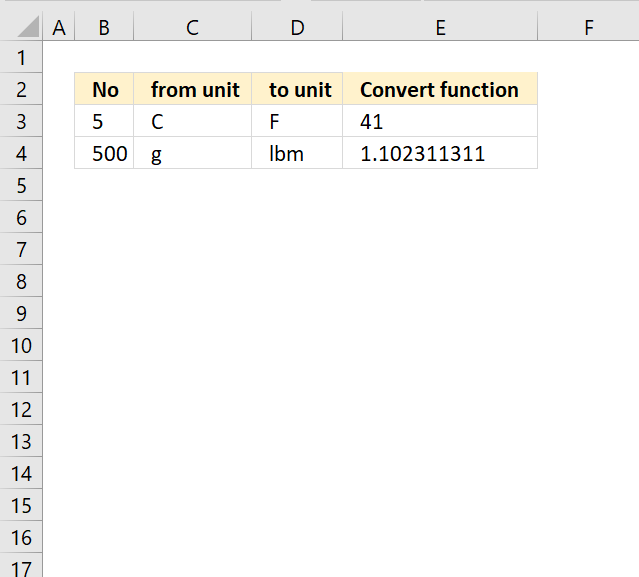Author: Oscar Cronquist Article last updated on March 29, 2018The CONVERT function converts a number from one measurement system to another.

Formula in cell E3:

=CONVERT(B3, C3, D3)

The CONVERT function converts from Celsius to Fahrenheit in cell E3.

### Excel Function Syntax

CONVERT(number, from_unit, to_unit)

### Arguments

 number Required. The number you want to convert from. from_unit Required. The unit you want to convert from. to_unit Required. The unit you want to convert to.
Weight and mass From_unit or to_unit
Gram "g"
Slug "sg"
Pound mass (avoirdupois) "lbm"
U (atomic mass unit) "u"
Ounce mass (avoirdupois) "ozm"
Grain "grain"
U.S. (short) hundredweight "cwt" or "shweight"
Imperial hundredweight "uk_cwt" or "lcwt" ("hweight")
Stone "stone"
Ton "ton"
Imperial ton "uk_ton" or "LTON" ("brton")
Distance From_unit or to_unit
Meter "m"
Statute mile "mi"
Nautical mile "Nmi"
Inch "in"
Foot "ft"
Yard "yd"
Angstrom "ang"
Ell "ell"
Light-year "ly"
Parsec "parsec" or "pc"
Pica (1/72 inch) "Picapt" or "Pica"
Pica (1/6 inch) "pica"
U.S survey mile (statute mile) "survey_mi"
Time From_unit or to_unit
Year "yr"
Day "day" or "d"
Hour "hr"
Minute "mn" or "min"
Second "sec" or "s"
Pressure From_unit or to_unit
Pascal "Pa" (or "p")
Atmosphere "atm" (or "at")
mm of Mercury "mmHg"
PSI "psi"
Torr "Torr"
Force From_unit or to_unit
Newton "N"
Dyne "dyn" (or "dy")
Pound force "lbf"
Pond "pond"
Energy From_unit or to_unit
Joule "J"
Erg "e"
Thermodynamic calorie "c"
IT calorie "cal"
Electron volt "eV" (or "ev")
Horsepower-hour "HPh" (or "hh")
Watt-hour "Wh" (or "wh")
Foot-pound "flb"
BTU "BTU" (or "btu")
Power From_unit or to_unit
Horsepower "HP" (or "h")
Pferdestärke "PS"
Watt "W" (or "w")
Magnetism From_unit or to_unit
Tesla "T"
Gauss "ga"
Temperature From_unit or to_unit
Degree Celsius "C" (or "cel")
Degree Fahrenheit "F" (or "fah")
Kelvin "K" (or "kel")
Degrees Rankine "Rank"
Degrees Réaumur "Reau"
Volume (or l iquid measure ) From_unit or to_unit
Teaspoon "tsp"
Modern teaspoon "tspm"
Tablespoon "tbs"
Fluid ounce "oz"
Cup "cup"
U.S. pint "pt" (or "us_pt")
U.K. pint "uk_pt"
Quart "qt"
Imperial quart (U.K.) "uk_qt"
Gallon "gal"
Imperial gallon (U.K.) "uk_gal"
Liter "l" or "L" ("lt")
Cubic angstrom "ang3" or "ang^3"
U.S. oil barrel "barrel"
U.S. bushel "bushel"
Cubic feet "ft3" or "ft^3"
Cubic inch "in3" or "in^3"
Cubic light-year "ly3" or "ly^3"
Cubic meter "m3" or "m^3"
Cubic Mile "mi3" or "mi^3"
Cubic yard "yd3" or "yd^3"
Cubic nautical mile "Nmi3" or "Nmi^3"
Cubic Pica "Picapt3", "Picapt^3", "Pica3" or "Pica^3"
Gross Registered Ton "GRT" ("regton")
Measurement ton (freight ton) "MTON"
Area From_unit or to_unit
International acre "uk_acre"
U.S. survey/statute acre "us_acre"
Square angstrom "ang2" or “ang^2"
Are "ar"
Square feet "ft2" or "ft^2"
Hectare "ha"
Square inches "in2" or "in^2"
Square light-year "ly2" or "ly^2"
Square meters "m2" or "m^2"
Morgen "Morgen"
Square miles "mi2" or "mi^2"
Square nautical miles "Nmi2" or "Nmi^2"
Square Pica "Picapt2", "Pica2", "Pica^2" or "Picapt^2"
Square yards "yd2" or "yd^2"
Information From_unit or to_unit
Bit "bit"
Byte "byte"
Speed From_unit or to_unit
Admiralty knot "admkn"
Knot "kn"
Meters per hour "m/h" or "m/hr"
Meters per second "m/s" or "m/sec"
Miles per hour "mph"
Prefix Multiplier Abbreviation
yotta 1E+24 "Y"
zetta 1E+21 "Z"
exa 1E+18 "E"
peta 1E+15 "P"
tera 1000000000000 "T"
giga 1000000000 "G"
mega 1000000 "M"
kilo 1000 "k"
hecto 100 "h"
dekao 10 "da" or "e"
deci 0.1 "d"
centi 0.01 "c"
milli 0.001 "m"
micro 0.000001 "u"
nano 0.000000001 "n"
pico 0.000000000001 "p"
femto 0.000000000000001 "f"
atto 1E-18 "a"
zepto 1E-21 "z"
yocto 1E-24 "y"
Binary Prefix Prefix Value Abbreviation Derived from
yobi 2^80 = 1 208 925 819 614 629 174 706 176 "Yi" yotta
zebi 2^70 = 1 180 591 620 717 411 303 424 "Zi" zetta
exbi 2^60 = 1 152 921 504 606 846 976 "Ei" exa
pebi 2^50 = 1 125 899 906 842 624 "Pi" peta
tebi 2^40 = 1 099 511 627 776 "Ti" tera
gibi 2^30 = 1 073 741 824 "Gi" giga
mebi 2^20 = 1 048 576 "Mi" mega
kibi 2^10 = 1024 "ki" kilo

### Comments

The CONVERT function returns a #N/A error if the unit doesn't exist.

Unit names and prefixes are case-sensitive.